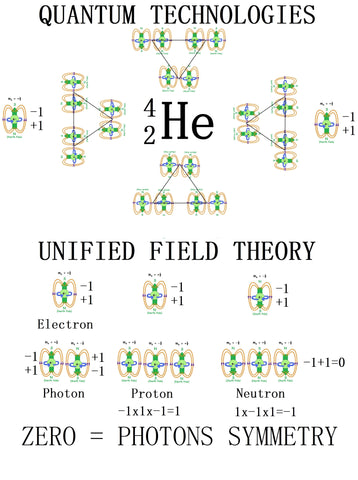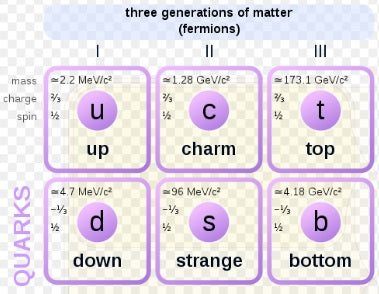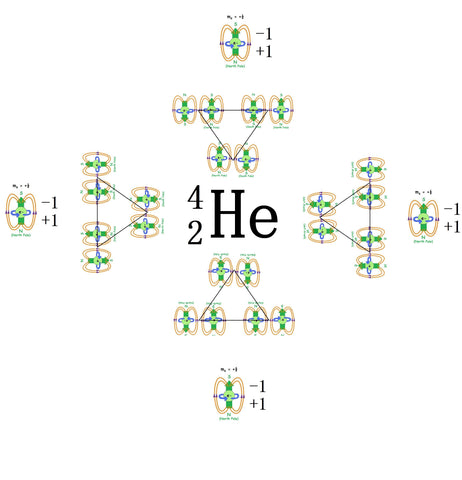# Symmetry in protons and neutrons and atomic balanceThe current standard model of physics used in chemistry has protons, neutrons and electrons but no positrons! Why? Well the positron was lost through the act of measuring which created the asymmetry in the structure of the atom. If the nucleus is just positive charges and the neutron is zero then how do they remain bound together? In my model the neutron is -1 and not 0. So where is my evidence for this hypothesis.

Neutron mass 1.67492749804(95)×10−27 kg
Mass of proton 1.67262192369(51)×10−27 kg
Mass of electron 9.1093837015(28)×10−31
Mass of positron 9.10938356(11)×10−31 kg

I propose the increased mass of the neutron is due to the positron and that is why the overall charge of the neutron is 0 and not -1.

Adding positrons back into the model for the periodic table and the implications of matter and anti-matter annellation. Understanding that the positron electron pair is a photon of light, which has no mass and no charge as they are identical and opposite in spin and charge therefore nothing is present because the cancel each other out but both exist in a symmetry state of opposites and half way between them is the nucleus (0,0,0) and Newtonian physics can be applied where r is 1/2 the wavelength of the photon. The idea that atoms contain electromagnetism is a useful concept and it is obtained when you rearrange Einstein's equation to c^2 = E/M where E/M equals rise / run on the mathematical cross.The geometry of light or electromagnetism where Einstein's equation creates the geometry of a sphere and the S orbital structure of hydrogen can be overlapped, where the concave and convex aspects of the sphere structure provides a lens to focus light into the center (nucleus) and inverts at this vanishing point to produce a symmetry of opposites. This is seen in the geometry of hydrogen wave functions, where the symmetry of opposites is observed.All of these different geometries have one thing in common and that is symmetry of opposites through a central point of 0,0,0 on a x,y,z geometry. Hence this symmetry has been lost in the 0 charge in the neutron.

So why do we have 6 quarks in the standard model?There is a symmetry of opposites. The neutron quarks form symmetry partners to the proton quarks as they can be considered electrons and positron pairs or photons. That is why the stay together in the nucleus as the one of the pairs is positive and the other negative and the balance of opposites maintained. The quarks up (in the SUSY inversion model are -1 and equivalent to an electron) and the down quark (in the SUSY inversion model is +1). Multiplication is used to obtain the charge. Neutron = -1 and the proton = +1. Overall charge of the paired neutron and proton is 0 providing stability. Quark up down pairs are photons. So the symmetry pairing is obvious when logic is used and not measurement. The added mass in the neutron which has been measured provides the smoking gun for the hidden anti-matter or the positron which has not been lost at all. Still present in every neutron but not understood previously. The three proton quarks match the three neutron quarks to make three photons of a given distance based on the atom and isotope.Positrons added to helium means 16 electrons are present in total. This is the key to unlock the unified field theory and consider an electron with a wavelength of two Planck lengths. This results in a mathematics that gives the correct ratios for dark matter and dark energy from alpha particle emission 13.8 billion light years ago. The previous standard model of physics cannot account for the missing antimatter because they think it has been eliminated rather than hidden by the act of measuring which creates the asymmetry in the model.

The positron or antielectron is the antiparticle or the antimatter counterpart of the electron. The positron has an electric charge of +1 e, a spin of 1/2 (the same as the electron), and has the same mass as an electron. When a positron collides with an electron, annihilation occurs. If this collision occurs at low energies, it results in the production of two or more photons. Rather than considering this as an annihilation and the loss of matter, consider the photon of no mass and no charge as the balance state of opposites in harmony where both state cancel each other out but both are present at a given distance which gives the wavelength and therefore the eV energy level. It is the tension between symmetry of 0 and the asymmetry of -1 and +1 that provides energy in the form of radicals, or short lived asymmetric states of photons, which are considered virtual particles that come in and out of existence. Noting that nothing is destroyed nor created but only transformed into symmetry (light) or asymmetry (physical form).

Radioactive decay and beta plus and beta minus.
I think the name gives it away or is it just too obvious for people to see? The beta plus decay going from the positive proton to the negative neutron and beta minus going from the negative neutron to the positive proton.
This is what Wiki state about beta decay.
In nuclear physicsbeta decay (β-decay) is a type of radioactive decay in which a beta particle (fast energetic electron or positron) is emitted from an atomic nucleus, transforming the original nuclide to an isobar of that nuclide. For example, beta decay of a neutron transforms it into a proton by the emission of an electron accompanied by an antineutrino; or, conversely a proton is converted into a neutron by the emission of a positron with a neutrino in so-called positron emission. Neither the beta particle nor its associated (anti-)neutrino exist within the nucleus prior to beta decay, but are created in the decay process.

If energy cannot be destroyed or created the concept of a positron hidden the neutron suggests that the beta particle is present in the atom and the asymmetry in the atom or the uneven number of protons and neutrons is responsible for the decay process in order to restore symmetry and stability back into the atom. If you consider the geometry of balanced opposites and the logic associated with the balance within the atom itself that has been lost through the act of measuring in order to make the atom visible then it would appear that measurement is flawed and this is the fundamental issue with quantum mechanics where the act of measurement is required to make the observation and what is observed is due to the measurement performed. As I have outlined above I have used a logical approach to determine that presence of anti-matter in the atomic model, this appears to be a feasible solution that has wide ranging implications.